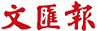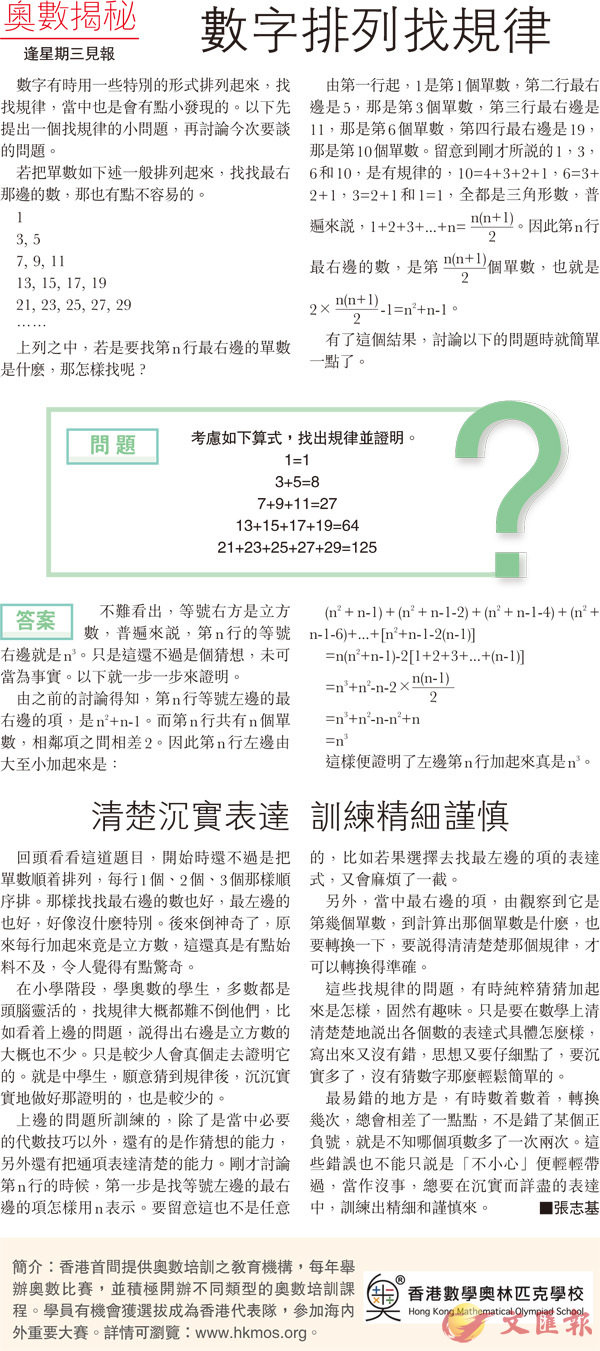首頁 > 文匯報 > 百科啟智 > 正文

【奧數揭秘】數字排列找規律

2017-10-181

3, 5

7, 9, 11

13, 15, 17, 19

21, 23, 25, 27, 29

......

1=1

3+5=8

7+9+11=27

13+15+17+19=64

21+23+25+27+29=125

(n2+n-1)+(n2+n-1-2)+(n2+n-1-4)+(n2+n-1-6)+...+[n2+n-1-2(n-1)]

=n(n2+n-1)-2[1+2+3+...+(n-1)]

=n3+n2-n-2×[n(n-1)]　

=n3+n2-n-n2+n

=n3